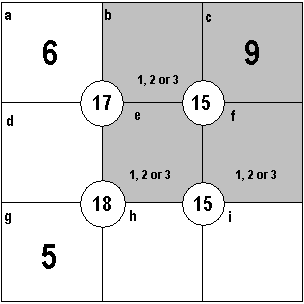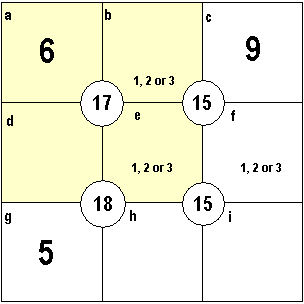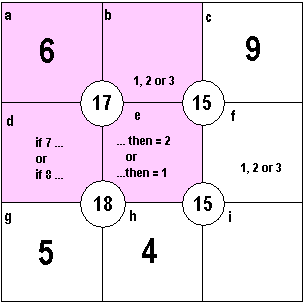summary:- tutorial to solve sujiko puzzles by the use of logic

# sujiko

These puzzles have just started in the Daily Telegraph and are another example of using pure logic to find the solution. You do not need to be good at maths.

As with all puzzles, a computer program can be written to solve it but where is the fun or skill in that? Only in writing the program - not in using it.

My first tutorial uses Sujiko puzzle #4 that appeared in the Daily Telegraph on March 29th. 2011 and rated with a 'moderate' level of difficulty.

The rules of Sujiko are simple. There is a 3x3 grid for the numbers 1 to 9; the position of some of them is given. Numbers can not be repeated and all the numbers 1 to 9 must be used.

In the grid are four circles containing a number. The number in each circle is the sum of the numbers in the four squares surrounding it.

My method is to take each block of four squares and list the possible numbers in each square and then use logic to get the result. As is often the case in tutorials, it takes a lot longer to explain here than to actually do it.

Here are some tips to help you work out a sujiko grid.Here is the Sujiko puzzle. 5, 6 and 9 are given so we are looking for the position of numbers 1, 2, 3, 4, 7 and 8 At a quick glance, the top right block seems to be a good starting point because 15 - 9 = 6 and we are looking for three different numbers that add up to six - and there are only three that will do that, namely 1 plus 2 plus 3 So we now know that each box b, e and f must be a 1 or a 2 or a 3 It therefore follows that boxes d, h and i cannot be 1, 2 or 3 and therefore must be a 4 or a 7 or an 8Now let us consider the top left block and find if box d is a 4, 7 or 8 working through the variables in box b and e d=17-6-1-2 = 8 or d=17-6-1-3 = 7 or d=17-6-2-3 = 6 = not possible as box 'a' is 6 so box d must be 7 or 8 and is not 4 It therefore follows that box h or i must be 4 Let us move on and look at the bottom left blockIf d = 7 or 8 and e = 1, 2 or 3 then the possibilities for h are... h=18-5-7-1 = 5 = not possible as g is 5 h=18-5-7-2 = 4 h=18-5-7-3 = 3 = not possible, only b, e or f=3 h=18-5-8-1 = 4 h=18-5-8-2 = 3 = not possible, only b, e or f=3 h=18-5-8-3 = 2 = not possible, only b, e or f=2 therefore box h must be 4 and, when d = 7 then e = 2 or, when d = 8 then e = 1 and, box e cannot be 3, only 1 or 2 That taught us a lot!Briefly, going back to the top left block we can now find the value of b because we know that the sum of d and e is 9, whatever the combination. So b = 17-6-9 = 2 If b = 2 then e must equal 1 If e = 1 and b = 2 then f must equal 3 If e = 1 then d must equal 8 Only one number left for box i and that is 7The solution. * Here are some tips for solving a sujiko grid. *